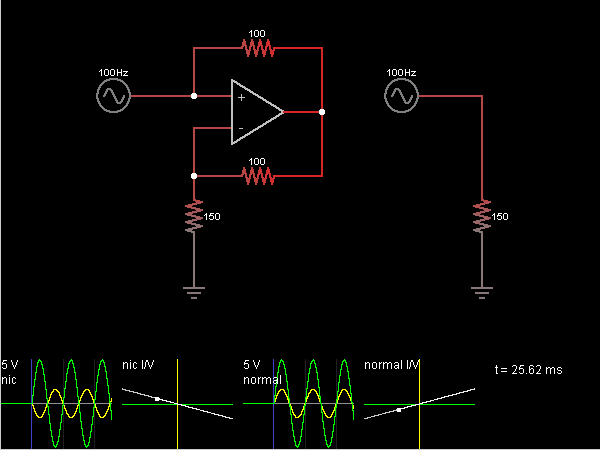# Circuit Simulator - Negative Impedance Converter

## Where can I get Negative Impedance Converter Circuit Diagram with Explanation?

IndiaBIX provides you lots of fully solved Negative Impedance Converter circuit diagram with detailed explanation and working principles.

## How to design a Negative Impedance Converter (electronic circuit)?

You can easily design the Negative Impedance Converter circuit by practicing the exercises given below. Here you can design and simulate your own electronic circuits with this Online Circuit Designer and Simulator.### Circuit Description:

The circuit on the left converts a positive impedance to a negative impedance. So, for example, instead of Ohm's Law (E=IR) it causes a resistor to obey E=-IR. The circuit on the right shows a positive impedance (a 150 ohm resistor) for comparison.

The op-amp attempts to keep its – input at the same voltage as the + input, which is connected to the input signal. So the impedance being transformed (a 150 ohm resistor) responds as if it were connected directly to the input signal. Whatever current it needs is sourced by the op-amp and flows through the bottom 100 ohm resistor.

Since the + input is at the same voltage as the – input, the current and voltage drop across the top 100 ohm resistor must be the same as the bottom one. As a result, when the input voltage is positive, current is flowing into the input rather than out of it. The input current is the same as the current through the impedance, but in the opposite direction. -- Credits: Mr. Paul Falstad.